# ATILA Harmonic Analysis

This solver includes the modal analysis of elastic, piezoelectric, magnetostrictive structures, closed fluid domains, and closed hydroelastic systems. A unique variant of this modal analysis is also included and efficiently performs the computation of the resonance and antiresonance modes of piezoelectric and magnetostrictive structures, as well as the effective coupling coefficient. Results include normal frequencies and normal modes, and effective coupling coefficient.

A tonpilz transducer is simulated as an example with conditions as below. 2D axis-symmetry was used and radiating boundary was assigned on the water shell.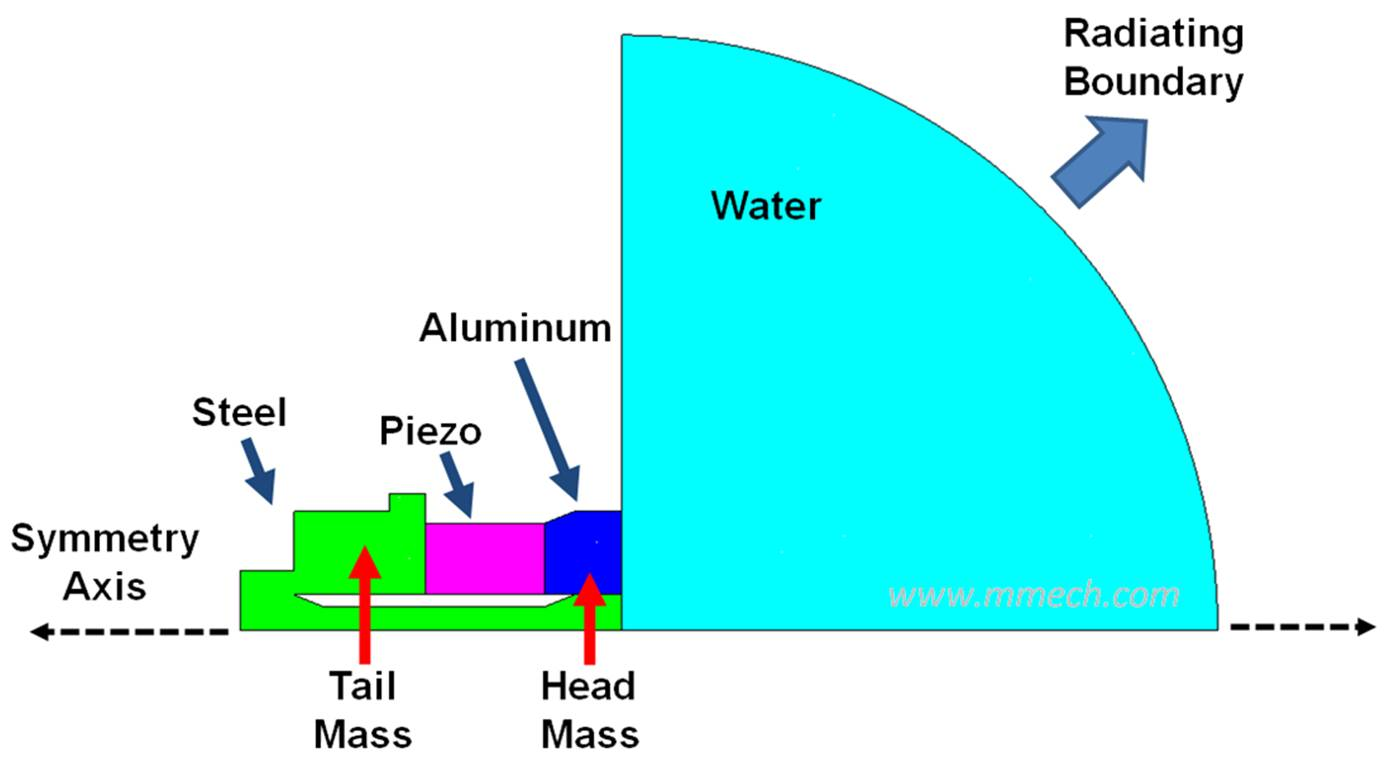Figure 1. A tonpilz transducer with ATILA.

ATILA harmonic analysis provides admittance, impedance, conductance, resistance, susceptance, and reactance graphs. Displacement, stress, fluid pressure, TVR, beam pattern, and Rp/Cp are also provided for the given frequency domain.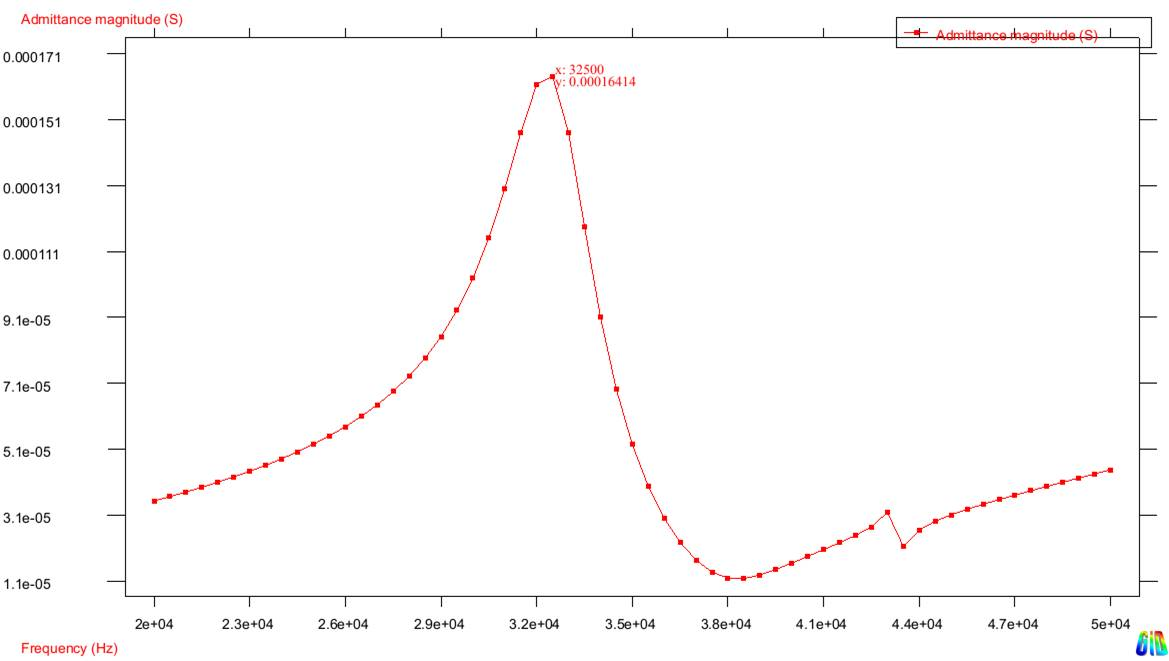Figure 2. Admittance graph from ATILA harmonic analysis.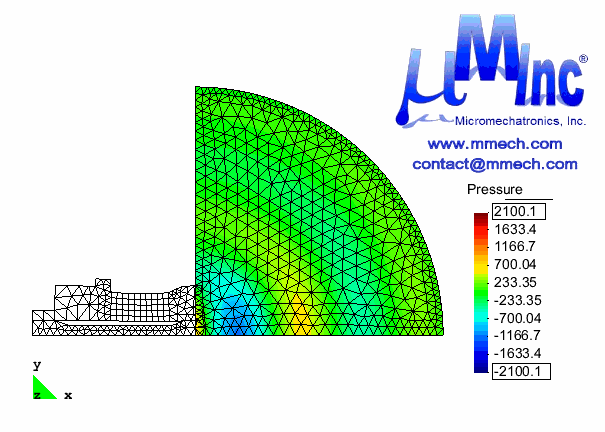Figure 3. Resonance mode at 32.5 kHz with fluid pressure analysis.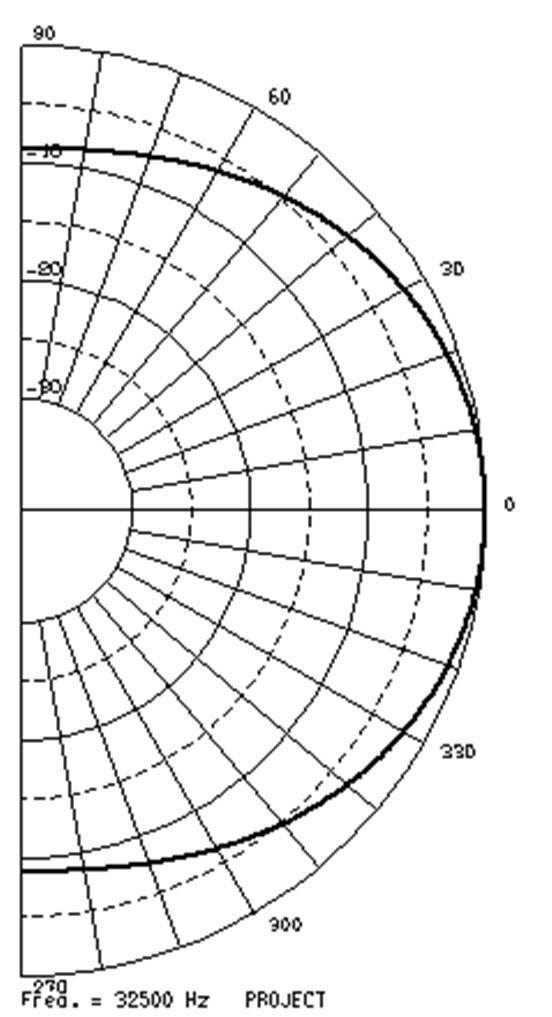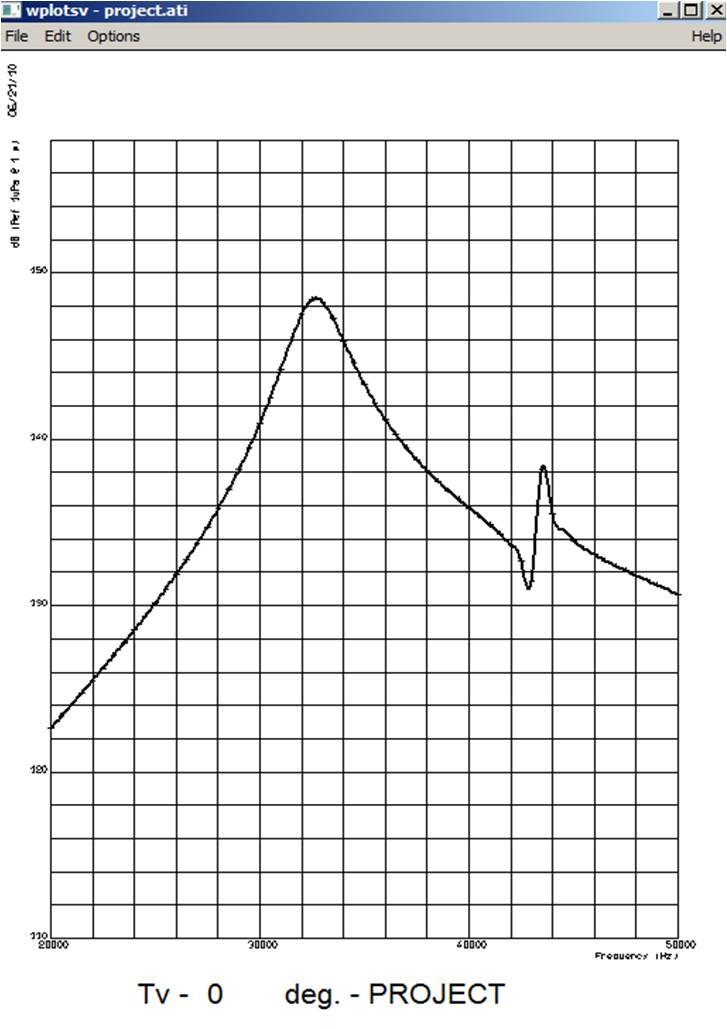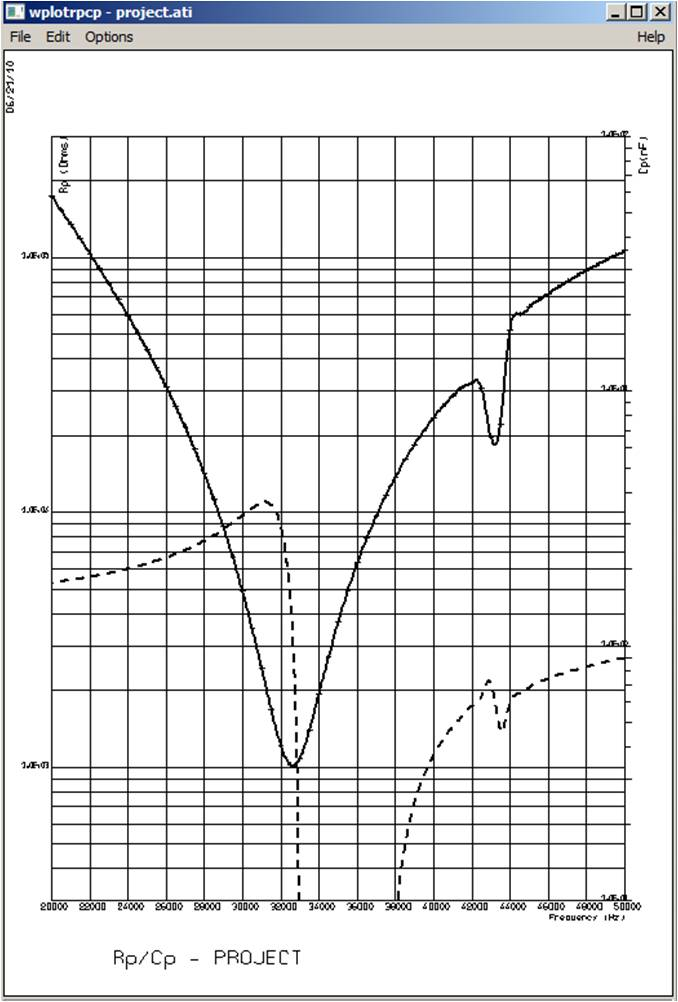Figure 4. Beam pattern, TVR, and Rp/Cp results from ATILA harmonic analysis